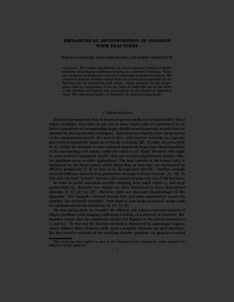Repository: Freie Universität Berlin, Math Department

# Hierarchical decomposition of domains with fractures

Gebauer, S. and Kornhuber, R. and Yserentant, H. (2006) Hierarchical decomposition of domains with fractures. Mathematics of Computation, 75 (253). pp. 73-90. ISSN 00255718Preview

173kB

Official URL: http://www.jstor.org/stable/4100144

## Abstract

We consider the efficient and robust numerical solution of elliptic problems with jumping coefficients occuring on a network of fractures. These thin geometric structures are resolved by anisotropic trapezoidal elements. We present an iterative solution concept based on a hierarchical separation of the fractures and the surrounding rock matrix. Upper estimates for the convergence rates are independent of the the jump of coefficients and of the width of the fractures and depend only polynomially on the number of refinement steps. The theoretical results are illustrated by numerical experiments.

Item Type: Article Mathematical and Computer Sciences > Mathematics > Numerical Analysis Department of Mathematics and Computer Science > Institute of Mathematics 1817 Ekaterina Engel 23 Feb 2016 09:54 03 Mar 2017 14:42

Repository Staff Only: item control page